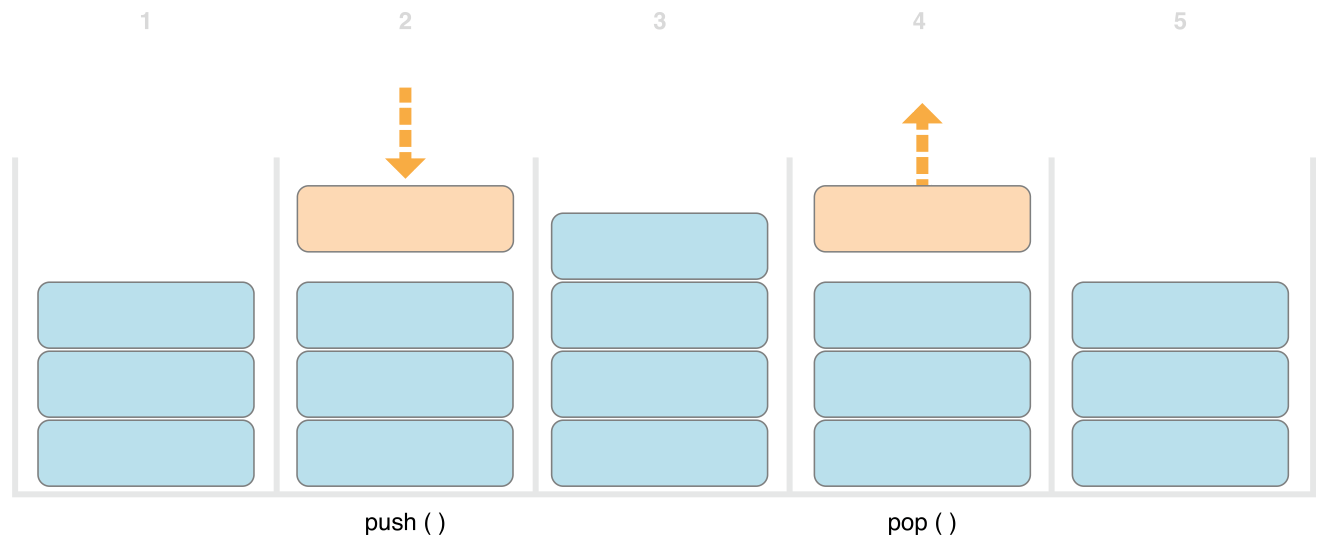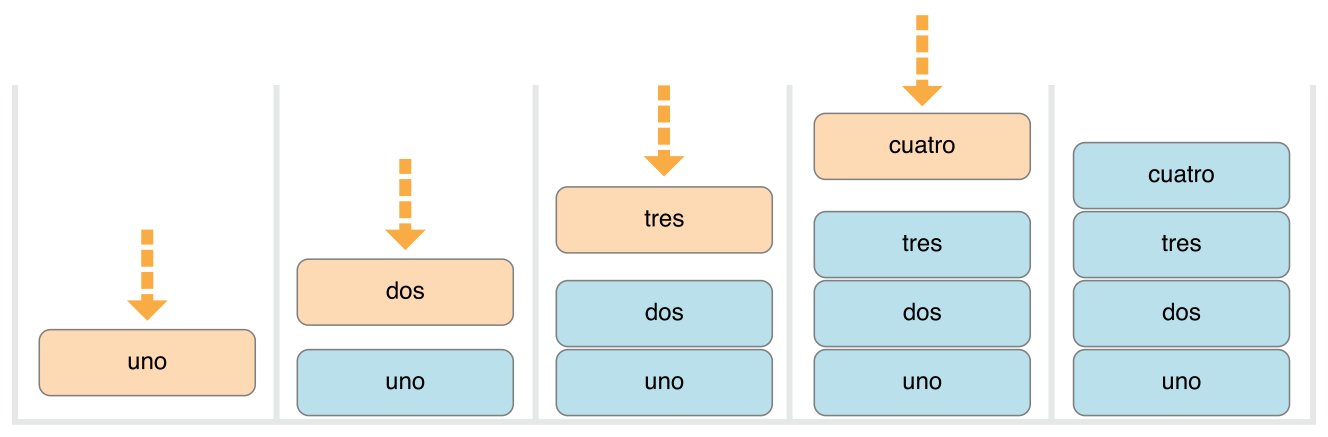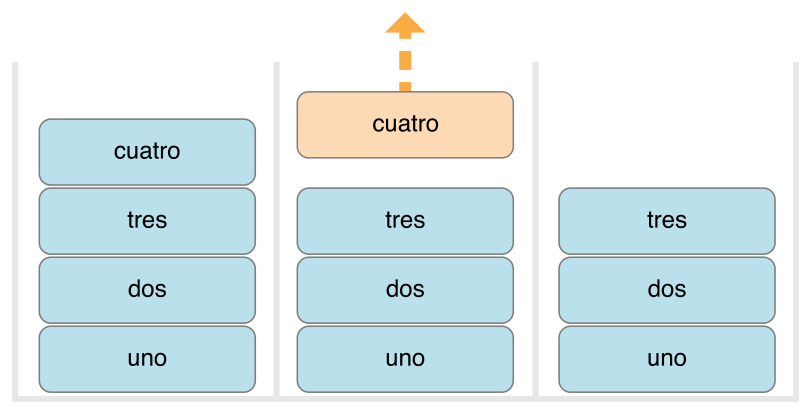# Swift学习之路-泛型

## 泛型解决的问题

``````func swapTwoInts(_ a: inout Int, _ b: inout Int) {
let temporaryA = a
a = b
b = temporaryA
}``````

`swapTwoInts(_:_:)` 函数把 b 原本的值给 a ，把 a 原本的值给 b 。你可以调用这个函数来交换两个 Int 变量的值。

``````var someInt = 3
var anotherInt = 107
swapTwoInts(&someInt, &anotherInt)
print("someInt is now \(someInt), and anotherInt is now \(anotherInt)")
// Prints "someInt is now 107, and anotherInt is now 3"``````

`swapTwoInts(_:_:)` 函数很实用，但是它只能用于 Int 值。如果你想交换两个 String 值，或者两个 Double 值，你只能再写更多的函数，比如下面的 `swapTwoStrings(_:_:)``swapTwoDoubles(_:_:)` 函数：

``````func swapTwoStrings(_ a: inout String, _ b: inout String) {
let temporaryA = a
a = b
b = temporaryA
}
func swapTwoDoubles(_ a: inout Double, _ b: inout Double) {
let temporaryA = a
a = b
b = temporaryA
}``````

## 泛型函数

``````func swapTwoValues<T>(_ a: inout T, _ b: inout T) {
let temporaryA = a
a = b
b = temporaryA
}``````

`swapTwoValues(_:_:)``swapTwoInts(_:_:)` 函数体是一样的。但是， `swapTwoValues(_:_:)``swapTwoInts(_:_:)` 的第一行有点不一样。下面是首行的对比：

``````func swapTwoInts(_ a: inout Int, _ b: inout Int)
func swapTwoValues<T>(_ a: inout T, _ b: inout T)``````

``````var someInt = 3
var anotherInt = 107
swapTwoValues(&someInt, &anotherInt)
// someInt is now 107, and anotherInt is now 3

var someString = "hello"
var anotherString = "world"
swapTwoValues(&someString, &anotherString)
// someString is now "world", and anotherString is now "hello"``````

## 泛型类型

UINavigationController 类在它的导航层级关系中管理视图控制器就是用的栈的思想。你可以调用 UINavigationController 类的 `pushViewController(_:animated:)` 方法添加（或者说push）一个视图控制器到导航栈里，用 `popViewControllerAnimated(_:)` 方法从导航栈移除（或者说pop）一个视图控制器。当你需要用严格的”后进，先出”方式管理一个集合时，栈是一个很有用的集合模型。1. 现在栈里有三个值；
2. 第四个值压到栈顶；
3. 栈里现在有四个值，最近添加的那个在顶部；
4. 栈中顶部的元素被移除，或者说叫”出栈”；
5. 移除一个元素之后，栈里又有三个元素了。

``````struct IntStack {
var items = [Int]()
mutating func push(_ item: Int) {
items.append(item)
}
mutating func pop() -> Int {
return items.removeLast()
}
}``````

``````struct Stack<Element> {
var items = [Element]()
mutating func push(_ item: Element) {
items.append(item)
}
mutating func pop() -> Element {
return items.removeLast()
}
}``````

Element 为稍后提供的”某类型 Element “定义了一个占位符名称。这个未来的类型可以在结构体定义内部任何位置以” Element “引用。在这个例子中，有三个地方将 Element 作为一个占位符使用：

• 创建一个名为 items 的属性，用一个 Element 类型值的空数组初始化这个属性；
• 指定 `push(_:)` 方法有一个叫做 item 的形式参数，其必须是 Element 类型；
• 指定 `pop() `方法的返回值是一个 Element 类型的值。

``````var stackOfStrings = Stack<String>()
stackOfStrings.push("uno")
stackOfStrings.push("dos")
stackOfStrings.push("tres")
stackOfStrings.push("cuatro")
// the stack now contains 4 strings````````````let fromTheTop = stackOfStrings.pop()
// fromTheTop is equal to "cuatro", and the stack now contains 3 strings``````## 扩展一个泛型类型

``````extension Stack {
var topItem: Element? {
return items.isEmpty ? nil : items[items.count - 1]
}
}``````

topItem 属性返回一个 Element 类型的可选值。如果栈是空的， topItem 返回 nil ；如果栈非空， topItem 返回 items 数组的最后一个元素。

``````if let topItem = stackOfStrings.topItem {
print("The top item on the stack is \(topItem).")
}
// Prints "The top item on the stack is tres."``````

## 类型约束

`swapTwoValues(_:_:)` 函数和 Stack 类型可以用于任意类型。但是，有时在用于泛型函数的类型和泛型类型上，强制其遵循特定的类型约束很有用。类型约束指出一个类型形式参数必须继承自特定类，或者遵循一个特定的协议、组合协议。

### 类型约束语法

``````func someFunction<T: SomeClass, U: SomeProtocol>(someT: T, someU: U) {
// function body goes here
}``````

### 类型约束的应用

``````func findIndex(ofString valueToFind: String, in array: [String]) -> Int? {
for (index, value) in array.enumerated() {
if value == valueToFind {
return index
}
}
return nil
}``````

`findIndex(ofString:in:)` 函数可以用于字符串数组中查找字符串值：

``````let strings = ["cat", "dog", "llama", "parakeet", "terrapin"]
if let foundIndex = findIndex(ofString: "llama", in: strings) {
print("The index of llama is \(foundIndex)")
}
// Prints "The index of llama is 2"``````

``````func findIndex<T>(of valueToFind: T, in array:[T]) -> Int? {
for (index, value) in array.enumerated() {
if value == valueToFind {
return index
}
}
return nil
}``````

``````func findIndex<T: Equatable>(of valueToFind: T, in array:[T]) -> Int? {
for (index, value) in array.enumerated() {
if value == valueToFind {
return index
}
}
return nil
}``````

`findIndex(of:in:)` 的类型形式参数写作 T: Equatable ，表示”任何遵循 Equatable 协议的类型 T “。

`findIndex(of:in:)` 函数现在可以成功编译，并且可以用于任何 Equatable 的类型，比如 Double 或者 String ：

``````let doubleIndex = findIndex(of: 9.3, in: [3.14159, 0.1, 0.25])
// doubleIndex is an optional Int with no value, because 9.3 is not in the array
let stringIndex = findIndex(of: "Andrea", in: ["Mike", "Malcolm", "Andrea"])
// stringIndex is an optional Int containing a value of 2``````

## 关联类型

### 关联类型的应用

``````protocol Container {
associatedtype ItemType
mutating func append(_ item: ItemType)
var count: Int { get }
subscript(i: Int) -> ItemType { get }
}``````

Container 协议定义了三个所有容器必须提供的功能：

• 必须能够通过 `append(_:)` 方法向容器中添加新元素；
• 必须能够通过一个返回 Int 值的 count 属性获取容器中的元素数量；
• 必须能够通过 Int 索引值的下标取出容器中每个元素。

``````struct IntStack: Container {
// original IntStack implementation
var items = [Int]()
mutating func push(_ item: Int) {
items.append(item)
}
mutating func pop() -> Int {
return items.removeLast()
}
// conformance to the Container protocol
typealias ItemType = Int
mutating func append(_ item: Int) {
self.push(item)
}
var count: Int {
return items.count
}
subscript(i: Int) -> Int {
return items[i]
}
}``````

IntStack 实现了 Container 协议所有的要求，为满足这些要求，封装了 IntStack 里现有的方法。

``````struct Stack<Element>: Container {
// original Stack<Element> implementation
var items = [Element]()
mutating func push(_ item: Element) {
items.append(item)
}
mutating func pop() -> Element {
return items.removeLast()
}
// conformance to the Container protocol
mutating func append(_ item: Element) {
self.push(item)
}
var count: Int {
return items.count
}
subscript(i: Int) -> Element {
return items[i]
}
}``````

### 给关联类型添加约束

``````protocol Container {
associatedtype Item: Equatable
mutating func append(_ item: Item)
var count: Int { get }
subscript(i: Int) -> Item { get }
}``````

### 在关联类型约束里使用协议

``````protocol SuffixableContainer: Container {
associatedtype Suffix: SuffixableContainer where Suffix.Item == Item
func suffix(_ size: Int) -> Suffix
}``````

``````extension Stack: SuffixableContainer {
func suffix(_ size: Int) -> Stack {
var result = Stack()
for index in (count-size)..<count {
result.append(self[index])
}
return result
}
// Inferred that Suffix is Stack.
}
var stackOfInts = Stack<Int>()
stackOfInts.append(10)
stackOfInts.append(20)
stackOfInts.append(30)
let suffix = stackOfInts.suffix(2)
// suffix contains 20 and 30``````

``````extension IntStack: SuffixableContainer {
func suffix(_ size: Int) -> Stack<Int> {
var result = Stack<Int>()
for index in (count-size)..<count {
result.append(self[index])
}
return result
}
// Inferred that Suffix is Stack<Int>.
}``````

### 扩展现有类型来指定关联类型

Swift 的 Array 类型已经提供了 `append(_:)` 方法、 count 属性、用 Int 索引取出其元素的下标。这三个功能满足了 Container 协议的要求。这意味着你可以通过简单地声明 Array 采纳协议，扩展 Array 使其遵循 Container 协议。通过一个空的扩展实现，如使用扩展声明采纳协议

``extension Array: Container {}``

## 泛型Where分句

``````func allItemsMatch<C1: Container, C2: Container>
(_ someContainer: C1, _ anotherContainer: C2) -> Bool
where C1.ItemType == C2.ItemType, C1.ItemType: Equatable {

// Check that both containers contain the same number of items.
if someContainer.count != anotherContainer.count {
return false
}

// Check each pair of items to see if they are equivalent.
for i in 0..<someContainer.count {
if someContainer[i] != anotherContainer[i] {
return false
}
}

// All items match, so return true.
return true
}``````

• C1 必须遵循 Container 协议（写作 C1: Container ）；
• C2 也必须遵循 Container 协议（写作 C2: Container ）；
• C1 的 ItemType 必须和 C2 的 ItemType 相同（写作 C1.ItemType == C2.ItemType ）；
• C1 的 ItemType 必须遵循 Equatable 协议（写作 C1.ItemType: Equatable ）。

• someContainer 是一个 C1 类型的容器；
• anotherContainer 是一个 C2 类型的容器；
• someContainer 和 anotherContainer 中的元素类型相同；
• someContainer 中的元素可以通过不等操作符（ != ）检查它们是否不一样。

`allItemsMatch(_:_:)` 函数开始会先检查两个容器中的元素数量是否相同。如果它们的元素数量不同，它们不可能匹配，函数就会返回 false 。

``````var stackOfStrings = Stack<String>()
stackOfStrings.push("uno")
stackOfStrings.push("dos")
stackOfStrings.push("tres")

var arrayOfStrings = ["uno", "dos", "tres"]

if allItemsMatch(stackOfStrings, arrayOfStrings) {
print("All items match.")
} else {
print("Not all items match.")
}
// Prints "All items match."``````

## 带有泛型 Where 分句的扩展

``````extension Stack where Element: Equatable {
func isTop(_ item: Element) -> Bool {
guard let topItem = items.last else {
return false
}
}
}``````

``````if stackOfStrings.isTop("tres") {
print("Top element is tres.")
} else {
print("Top element is something else.")
}
// Prints "Top element is tres."``````

``````struct NotEquatable { }
var notEquatableStack = Stack<NotEquatable>()
let notEquatableValue = NotEquatable()
notEquatableStack.push(notEquatableValue)
notEquatableStack.isTop(notEquatableValue) // Error``````

``````extension Container where Item: Equatable {
func startsWith(_ item: Item) -> Bool {
return count >= 1 && self == item
}
}``````

`startsWith(_:)` 方法首先确保容器拥有至少一个元素，然后它检查第一个元素是否与给定元素相同。这个新的 `startsWith(_:)` 方法可以应用到任何遵循 Container 协议的类型上，包括前面我们用的栈和数组，只要容器的元素可以判等。

``````if [9, 9, 9].startsWith(42) {
print("Starts with 42.")
} else {
print("Starts with something else.")
}
// Prints "Starts with something else."``````

``````extension Container where Item == Double {
func average() -> Double {
var sum = 0.0
for index in 0..<count {
sum += self[index]
}
return sum / Double(count)
}
}
print([1260.0, 1200.0, 98.6, 37.0].average())
// Prints "648.9"``````

## 上下文 Where 分句

``````extension Container {
func average() -> Double where Item == Int {
var sum = 0.0
for index in 0..<count {
sum += Double(self[index])
}
return sum / Double(count)
}
func endsWith(_ item: Item) -> Bool where Item: Equatable {
return count >= 1 && self[count-1] == item
}
}
let numbers = [1260, 1200, 98, 37]
print(numbers.average())
// Prints "648.75"
print(numbers.endsWith(37))
// Prints "true"``````

``````extension Container where Item == Int {
func average() -> Double {
var sum = 0.0
for index in 0..<count {
sum += Double(self[index])
}
return sum / Double(count)
}
}
extension Container where Item: Equatable {
func endsWith(_ item: Item) -> Bool {
return count >= 1 && self[count-1] == item
}
}``````

## 关联类型的泛型 Where 分句

``````protocol Container {
associatedtype Item
mutating func append(_ item: Item)
var count: Int { get }
subscript(i: Int) -> Item { get }

associatedtype Iterator: IteratorProtocol where Iterator.Element == Item
func makeIterator() -> Iterator
}``````

Iterator 中的泛型 where 分句要求遍历器以相同的类型遍历容器内的所有元素，无论遍历器是什么类型。 `makeIterator()` 函数提供了容器的遍历器的访问。

``protocol ComparableContainer: Container where Item: Comparable { }``

## 泛型下标

``````extension Container {
subscript<Indices: Sequence>(indices: Indices) -> [Item]
where Indices.Iterator.Element == Int {
var result = [Item]()
for index in indices {
result.append(self[index])
}
return result
}
}``````

• 在尖括号中的泛型形式参数 Indices 必须是遵循标准库中 Sequence 协议的某类型；
• 下标接收单个形式参数， indices ，它是一个 Indices 类型的实例；
• 泛型 where 分句要求序列的遍历器必须遍历 Int 类型的元素。这就保证了序列中的索引都是作为容器索引的相同类型。